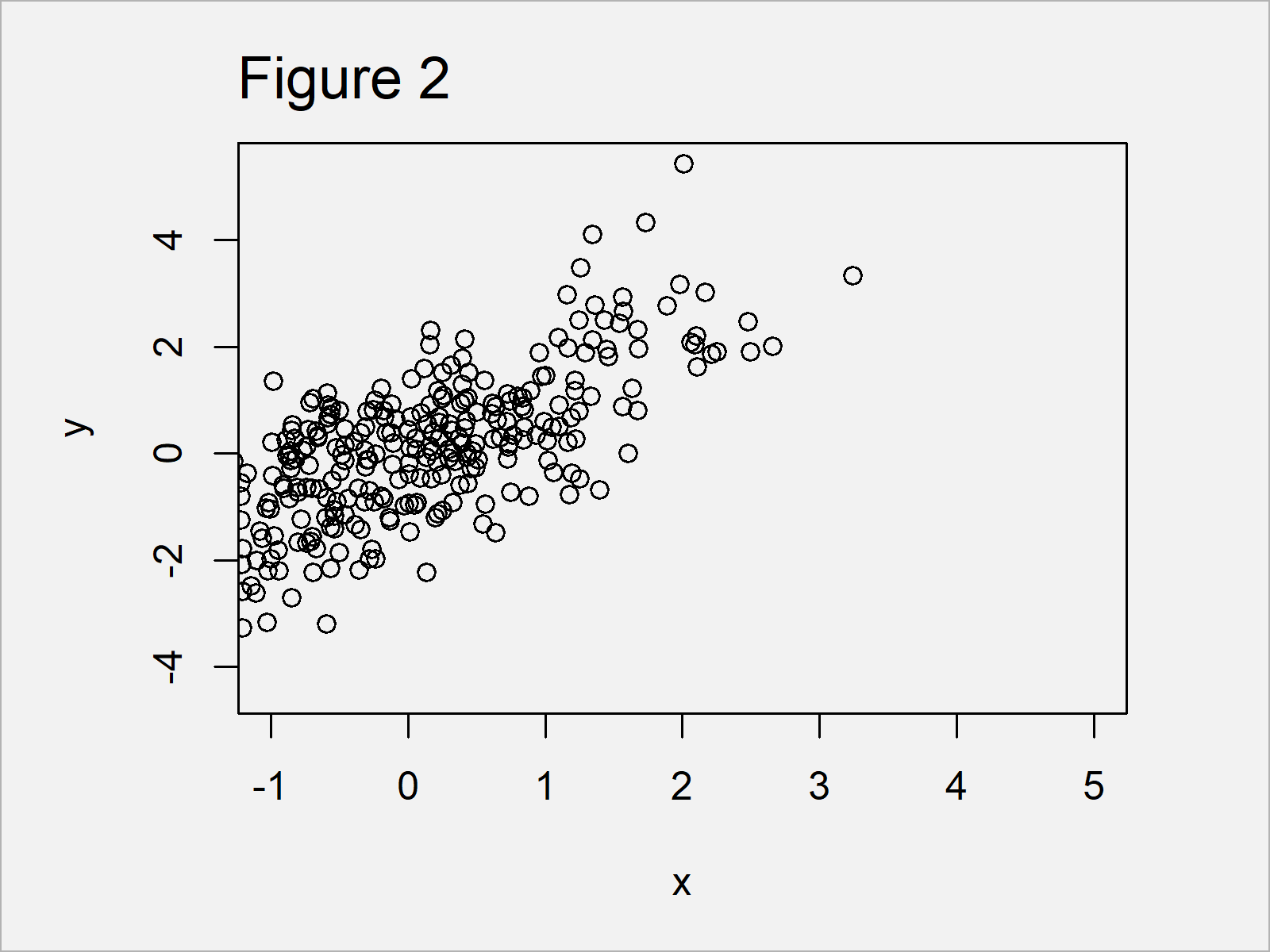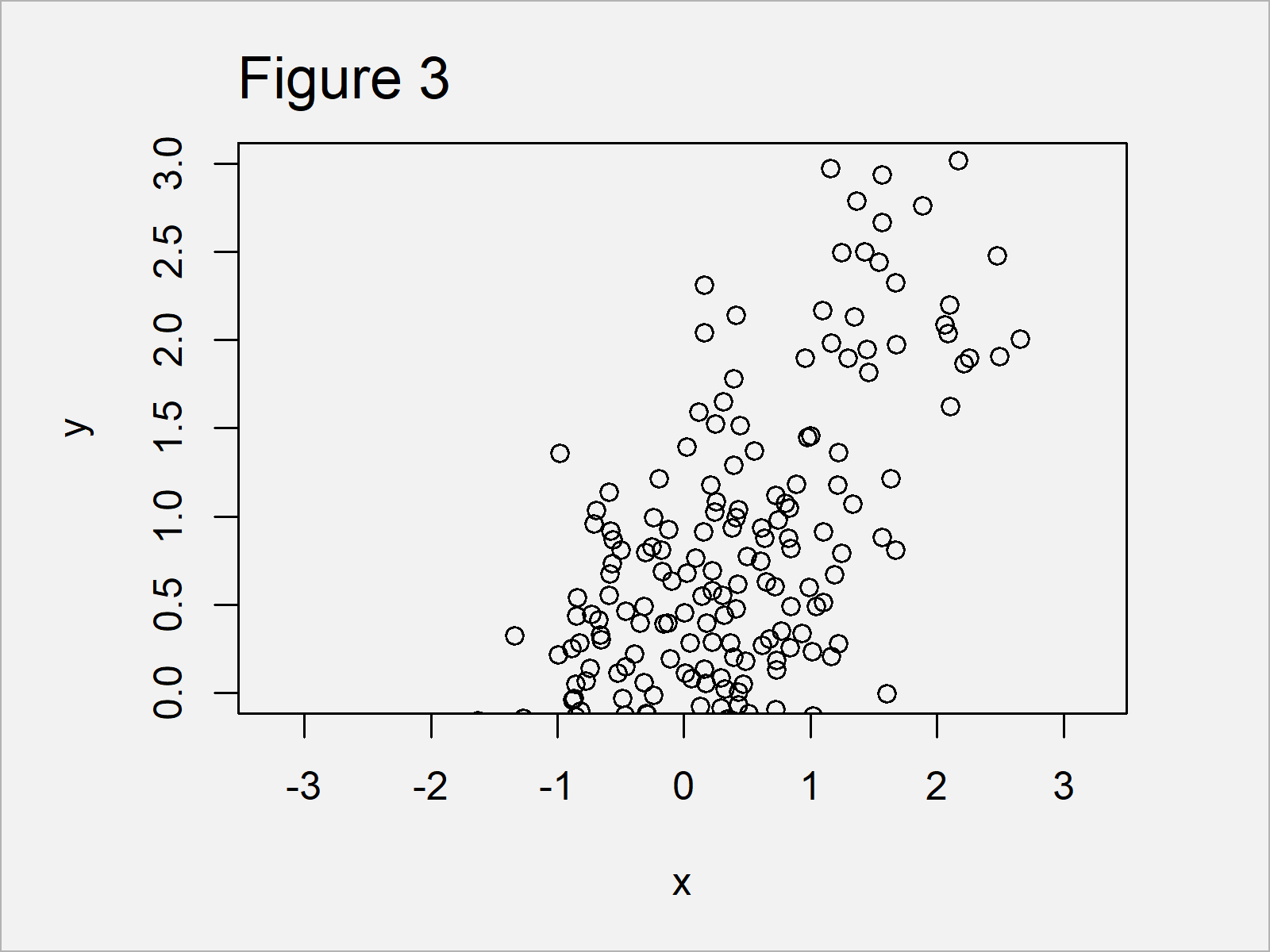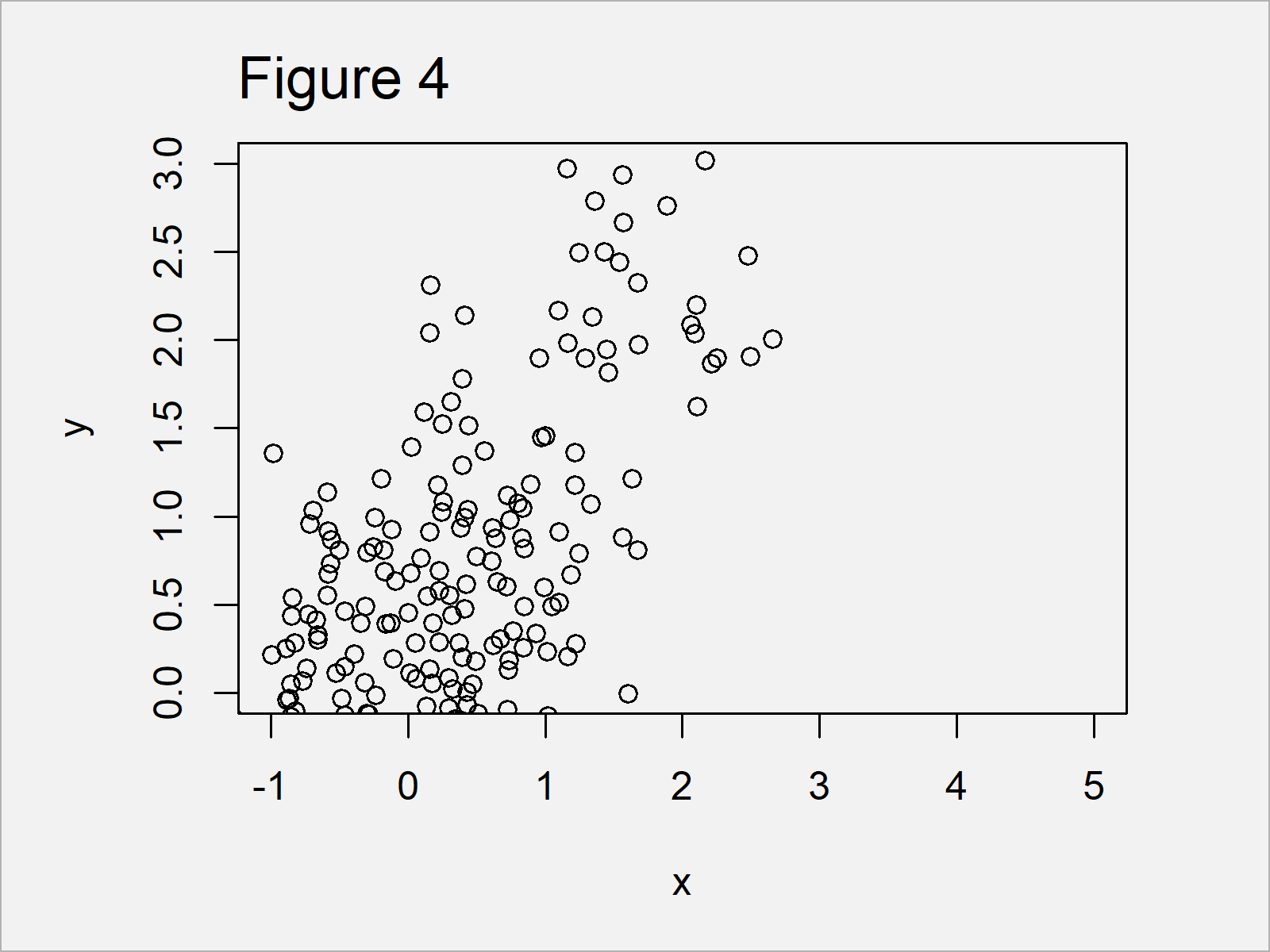# Set Axis Limits of Plot in R (3 Examples)

In this tutorial, I’ll explain how to change x-axis and y-axis limits in the R programming language.

The article is structured as follows:

With that, let’s do this!

## Creation of Example Data

As the first step, I’ll need to construct some data that we can use in the example syntax later on:

```set.seed(259687)                     # Create example data
x <- rnorm(300)
y <- rnorm(300) + x```

The previously shown R code has created two randomly distributed numeric vectors.

Now, we can create a graph of our data as follows:

`plot(x, y)                           # Draw example data with default axes`As shown in Figure 1, the previous R programming code has managed to create a scatterplot by applying the plot() function to our two numeric vectors.

The x- and y-axis limits have been chosen automatically based on the minimum and maximum values in our data.

In the following examples, I’ll explain how to adjust the axis limits of our graphic manually.

## Example 1: Set X-Axis Limits Using xlim Argument

Example 1 shows how to change the x-axis limits of our graph using the xlim argument.

As you can see in the following R code, we have to assign a vector consisting of two numeric values to the xlim argument.

The first value specifies the lower axis limit and the second value specifies the upper axis limit.

Let’s run the following R code:

```plot(x, y,                           # Apply xlim argument
xlim = c(- 1, 5))```By running the previous syntax we have created Figure 2, i.e. a scatterplot with a manually specified x-axis range.

The y-axis range, however, has still been selected automatically by the R programming language.

## Example 2: Set Y-Axis Limits Using ylim Argument

The following R programming syntax explains how to use the ylim argument to set the y-axis limits in R.

Similar as in case of the xlim option (shown in Example 1), we have to assign a vector with a length of two to the ylim argument.

Consider the following R syntax:

```plot(x, y,                           # Apply ylim argument
ylim = c(0, 3))```As shown in Figure 3, we have drawn another xy-plot with the previous R code. However, this time the y-axis limits were set by the user and the x-axis were set to the default values.

## Example 3: Set X-Axis & Y-Axis Limits Using xlim & ylim Arguments Simultaneously

The following R code explains how to change the x-axis and y-axis limits of our plot at the same time.

For this task, we have to specify both the xlim and ylim arguments:

```plot(x, y,                           # Apply xlim & ylim arguments
xlim = c(- 1, 5),
ylim = c(0, 3))```In Figure 4 it is shown that we have drawn a dotplot where all axes have been defined manually.

## Video, Further Resources & Summary

Have a look at the following video which I have published on my YouTube channel. In the video, I illustrate the R programming syntax of this article.

Please accept YouTube cookies to play this video. By accepting you will be accessing content from YouTube, a service provided by an external third party.If you accept this notice, your choice will be saved and the page will refresh.

At this point you should know how to manually adjust axis limits using xlim and ylim in R.

In the present tutorial, we have changed the axis limits of a scatterplot. However, please note that we could apply the same type of code to change the axes of other graphs such as barcharts, boxplots, and line plots as well.

If you have further questions, let me know in the comments section below.

Subscribe to the Statistics Globe Newsletter

•James White
April 30, 2023 6:20 pm

Very helpful. Is it the same for scatterplots? And what about R? Scatterplot, boxplot?

•May 2, 2023 8:57 am